# Resources tagged with: Curious

Filter by: Content type:
Age range:
Challenge level:

### There are 68 results

Broad Topics > Habits of Mind > Curious### Charlie's Delightful Machine

##### Age 11 to 16 Challenge Level:

Here is a machine with four coloured lights. Can you develop a strategy to work out the rules controlling each light?### More Number Pyramids

##### Age 11 to 14 Challenge Level:

When number pyramids have a sequence on the bottom layer, some interesting patterns emerge...### Stars

##### Age 11 to 14 Challenge Level:

Can you find a relationship between the number of dots on the circle and the number of steps that will ensure that all points are hit?### What Numbers Can We Make?

##### Age 11 to 14 Challenge Level:

Imagine we have four bags containing a large number of 1s, 4s, 7s and 10s. What numbers can we make?### Multiplication Arithmagons

##### Age 14 to 16 Challenge Level:

Can you find the values at the vertices when you know the values on the edges of these multiplication arithmagons?### Number Pyramids

##### Age 11 to 14 Challenge Level:

Try entering different sets of numbers in the number pyramids. How does the total at the top change?### Arithmagons

##### Age 11 to 16 Challenge Level:

Can you find the values at the vertices when you know the values on the edges?### Elevenses

##### Age 11 to 14 Challenge Level:

How many pairs of numbers can you find that add up to a multiple of 11? Do you notice anything interesting about your results?### Square Coordinates

##### Age 11 to 14 Challenge Level:

A tilted square is a square with no horizontal sides. Can you devise a general instruction for the construction of a square when you are given just one of its sides?### What Numbers Can We Make Now?

##### Age 11 to 14 Challenge Level:

Imagine we have four bags containing numbers from a sequence. What numbers can we make now?### Summing Consecutive Numbers

##### Age 11 to 14 Challenge Level:

15 = 7 + 8 and 10 = 1 + 2 + 3 + 4. Can you say which numbers can be expressed as the sum of two or more consecutive integers?### Shifting Times Tables

##### Age 11 to 14 Challenge Level:

Can you find a way to identify times tables after they have been shifted up or down?### Pair Products

##### Age 14 to 16 Challenge Level:

Choose four consecutive whole numbers. Multiply the first and last numbers together. Multiply the middle pair together. What do you notice?### Nice or Nasty

##### Age 7 to 14 Challenge Level:

There are nasty versions of this dice game but we'll start with the nice ones...### Partly Painted Cube

##### Age 14 to 16 Challenge Level:

Jo made a cube from some smaller cubes, painted some of the faces of the large cube, and then took it apart again. 45 small cubes had no paint on them at all. How many small cubes did Jo use?### Beelines

##### Age 14 to 16 Challenge Level:

Is there a relationship between the coordinates of the endpoints of a line and the number of grid squares it crosses?### Cuboid Challenge

##### Age 11 to 16 Challenge Level:

What's the largest volume of box you can make from a square of paper?### How Much Can We Spend?

##### Age 11 to 14 Challenge Level:

A country has decided to have just two different coins, 3z and 5z coins. Which totals can be made? Is there a largest total that cannot be made? How do you know?### Take Three from Five

##### Age 14 to 16 Challenge Level:

Caroline and James pick sets of five numbers. Charlie chooses three of them that add together to make a multiple of three. Can they stop him?### Semi-regular Tessellations

##### Age 11 to 16 Challenge Level:

Semi-regular tessellations combine two or more different regular polygons to fill the plane. Can you find all the semi-regular tessellations?### Marbles in a Box

##### Age 11 to 16 Challenge Level:

How many winning lines can you make in a three-dimensional version of noughts and crosses?### Searching for Mean(ing)

##### Age 11 to 16 Challenge Level:

If you have a large supply of 3kg and 8kg weights, how many of each would you need for the average (mean) of the weights to be 6kg?### On the Edge

##### Age 11 to 14 Challenge Level:

If you move the tiles around, can you make squares with different coloured edges?### Right Angles

##### Age 11 to 14 Challenge Level:

Can you make a right-angled triangle on this peg-board by joining up three points round the edge?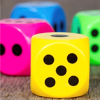### Dicey Operations

##### Age 11 to 14 Challenge Level:

Who said that adding, subtracting, multiplying and dividing couldn't be fun?### What's Possible?

##### Age 14 to 16 Challenge Level:

Many numbers can be expressed as the difference of two perfect squares. What do you notice about the numbers you CANNOT make?### Special Numbers

##### Age 11 to 14 Challenge Level:

My two digit number is special because adding the sum of its digits to the product of its digits gives me my original number. What could my number be?### A Little Light Thinking

##### Age 14 to 16 Challenge Level:

Here is a machine with four coloured lights. Can you make two lights switch on at once? Three lights? All four lights?### Satisfying Statements

##### Age 11 to 14 Challenge Level:

Can you find any two-digit numbers that satisfy all of these statements?### A Chance to Win?

##### Age 11 to 14 Challenge Level:

Imagine you were given the chance to win some money... and imagine you had nothing to lose...### Pick's Theorem

##### Age 14 to 16 Challenge Level:

Polygons drawn on square dotty paper have dots on their perimeter (p) and often internal (i) ones as well. Find a relationship between p, i and the area of the polygons.### Can They Be Equal?

##### Age 11 to 14 Challenge Level:

Can you find rectangles where the value of the area is the same as the value of the perimeter?### Largest Product

##### Age 11 to 14 Challenge Level:

Which set of numbers that add to 10 have the largest product?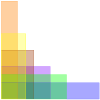### Perimeter Possibilities

##### Age 11 to 14 Challenge Level:

I'm thinking of a rectangle with an area of 24. What could its perimeter be?### Litov's Mean Value Theorem

##### Age 11 to 14 Challenge Level:

Start with two numbers and generate a sequence where the next number is the mean of the last two numbers...### Cosy Corner

##### Age 11 to 14 Challenge Level:

Six balls are shaken. You win if at least one red ball ends in a corner. What is the probability of winning?##### Age 11 to 14 Challenge Level:

Think of a number and follow my instructions. Tell me your answer, and I'll tell you what you started with! Can you explain how I know?### Two's Company

##### Age 11 to 14 Challenge Level:

Seven balls are shaken. You win if the two blue balls end up touching. What is the probability of winning?### Think of Two Numbers

##### Age 11 to 14 Challenge Level:

Think of two whole numbers under 10, and follow the steps. I can work out both your numbers very quickly. How?### Cola Can

##### Age 11 to 14 Challenge Level:

An aluminium can contains 330 ml of cola. If the can's diameter is 6 cm what is the can's height?### How Old Am I?

##### Age 14 to 16 Challenge Level:

In 15 years' time my age will be the square of my age 15 years ago. Can you work out my age, and when I had other special birthdays?### Sending a Parcel

##### Age 11 to 14 Challenge Level:

What is the greatest volume you can get for a rectangular (cuboid) parcel if the maximum combined length and girth are 2 metres?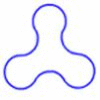### Arclets

##### Age 14 to 16 Challenge Level:

Each of the following shapes is made from arcs of a circle of radius r. What is the perimeter of a shape with 3, 4, 5 and n "nodes".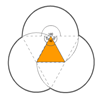### Triangles and Petals

##### Age 14 to 16 Challenge Level:

An equilateral triangle rotates around regular polygons and produces an outline like a flower. What are the perimeters of the different flowers?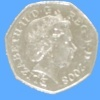### Last One Standing

##### Age 14 to 16 Challenge Level:

Imagine a room full of people who keep flipping coins until they get a tail. Will anyone get six heads in a row?### Which Spinners?

##### Age 14 to 18 Challenge Level:

Can you work out which spinners were used to generate the frequency charts?### Where to Land

##### Age 14 to 16 Challenge Level:

Chris is enjoying a swim but needs to get back for lunch. If she can swim at 3 m/s and run at 7m/sec, how far along the bank should she land in order to get back as quickly as possible?### Curvy Areas

##### Age 14 to 16 Challenge Level:

Have a go at creating these images based on circles. What do you notice about the areas of the different sections?### Triangle Midpoints

##### Age 14 to 16 Challenge Level:

You are only given the three midpoints of the sides of a triangle. How can you construct the original triangle?### What's it Worth?

##### Age 11 to 16 Challenge Level:

There are lots of different methods to find out what the shapes are worth - how many can you find?